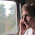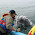## Friday, October 26, 2012

### Accessing vertices from a polygon with OGR/gdal and Python

It took me a bit to figure out how to access the single vertices of a polygon using gdal/ogr, but I found the missing key towards the answer finally on this page

The shapefile contains one or more layers. These layers again can contain one or more features, each having attributed and a geometry, which can be an polygon, for example. However, this geometry, being a polygon, contains another geometry, a "Linear Ring", and it is this geometry deep down I have to access to get individual points.

It's seen in the gml notation, where the polygon contains a Linear Ring:

     <gml:Polygon>          <gml:outerBoundaryIs>                  <gml:LinearRing>                          <gml:posList>0,0 100,0 100,100 0,100 0,0</gml:posList>                  </gml:LinearRing>         </gml:outerBoundaryIs>      </gml:Polygon> 

So how to dig down to this Linear Ring and thus the individual vertices?

How to open shapefiles is nicely described in lecture one on this very helpful page  :

     import ogr, sys, os     fn = 'C:/Users/max/Documents/GlacierMasks2000s_CryoClimMøte.shp'     driver = ogr.GetDriverByName('ESRI Shapefile')     dataSource = driver.Open(fn, 0) 

I can check how many layers my file contains:

     >>> dataSource.GetLayerCount()     1     >>> 

and since it is only one layer, I proceed to access this layer. Being one layer only, it has the index "0":

     >>> layer = dataSource.GetLayer(0) 

I can check how many features this layer contains:

     >>> layer.GetFeatureCount()     1560     >>>

For this example I only want to get one of the features, also checking some info about it. The item list is the attribute table entry for this feature:

>>> feature = layer.GetFeature(44)    >>> feature.GetFieldCount()    21    >>> feature.items()    {'Comment': None, 'Y_cent': 8869558.8657, 'SatelliteI': 'SPI 08-027    Svalbard', 'IDENT': 22210.0, 'OBJECTID_1': 45, 'NAME': 'Idunbreen', 'OBJECTID': 46, 'NumLines': 2, 'Shape_Area': 333164842.804, 'X_cent': 592561.435311, 'SOURCE2': 'SPIRIT-SPOT DEM, ASTER DEM (GDEM), NPI DEM, Unpubl SPRI Interferometric velocities', 'SOURCE': 'SPOT5HRS-IPY_SPIRIT_PROJECT (Korona et. al.,2009)', 'YEAR_': 2008, 'AvgLength': 24053.902741, 'DDMM': 1408, 'MaxLength': 25786.323167, 'New_additi': 0, 'Shape_Le_1': 87176.4155756, 'TIDEWATER': 1, 'ANALYST': 'Rickard Pettersson, Uppsala University, Sweden', 'Shape_Leng': 87176.415576} 

But I want to access the geometry in this feature, finding out if it is a point, a polygon or...

>>> geometry = feature.GetGeometryRef()    >>> geometry.GetGeometryCount()    1    >>> geometry.GetGeometryName()    'POLYGON'    >>>

At this point I thought, I already could access the vertices of the polygon, but this does not work:

>>> geometry.GetPointCount()   0   >>> geometry.GetX()   0.0   ERROR 6: Incompatible geometry for operation   >>>

I have to dig one level deeper and access the geometry contained within the polygon:

>>> ring = geometry.GetGeometryRef(0)   >>> ring.GetGeometryName()   'LINEARRING'   >>> ring.GetPointCount()   578   >>> ring.GetPoint(500)   (599109.9332999997, 8882494.9067, 0.0)   >>>

The last line is the latitude, longitude and elevation information for point number 500.

Finally a code collecting all of this and looping through the points and writing them into a text file:

 import ogr, os, sys # Open Shapefile fn = 'C:/Users/max/Documents/GlacierMasks2000s_CryoClimMøte.shp' driver = ogr.GetDriverByName('ESRI Shapefile') dataSource = driver.Open(fn, 0) # Get Layer print 'The file contains ', dataSource.GetLayerCount(), ' Layers' layer = dataSource.GetLayer(0) # Get Features print layer.GetName(), ' contains ', layer.GetFeatureCount(), ' features' feature = layer.GetFeature(44) # Get Geometry geometry = feature.GetGeometryRef() print ' Feature contains the Geometry', geometry.GetGeometryName() print ' It contains', geometry.GetGeometryCount(), geometry.GetGeometryName() # Get Geometry inside Geometry ring = geometry.GetGeometryRef(0) print geometry.GetGeometryName(), ' contains the Geometry', ring.GetGeometryName() print ' It contains', ring.GetPointCount(), ' points in a ', ring.GetGeometryName() # Write points in Vectors and Textfile pointsX = []; pointsY = []; pointsZ = [] numpoints = ring.GetPointCount() file = open(r'C:\Users\max\Documents\PythonProjects\text.txt','w') for p in range(numpoints):         lon, lat, z = ring.GetPoint(p)         pointsX.append(lon)         pointsY.append(lat)         pointsZ.append(z)         x = [str(lon), str(lat), str(z), '\n']         file.writelines(x)    print ' the first 5 points ', pointsX[0:10], pointsY[0:10] , pointsZ[0:10]       
dataSource = None
 file.close() #end 

#### Matlab comparison

In Matlab I can read the same shapefile with

>> shape = shaperead('C:/Users/max/Documents/GlacierMasks2000s_CryoClimMøte.shp')    shape =    1560x1 struct array with fields:     Geometry     BoundingBox     X     Y     OBJECTID_1     OBJECTID     NAME     Comment     IDENT     New_additi     YEAR_     DDMM     ANALYST     SOURCE     SOURCE2     SatelliteI     Shape_Leng     X_cent     Y_cent     TIDEWATER     MaxLength     AvgLength     NumLines     Shape_Le_1     Shape_Area

  To access the same polygon as in the example above, Matlab uses the following command. But be aware, Python counts the indices from "0" and Matlab from "1". THe "feature.item()" command in Python above shows the OBJECTID of 46 for feature 44, which in Matlab is feature 45:  >> shape(45).OBJECTID ans =     46 >> length( shape(45).X ) ans =    579 

The X and Y in the struct array corresponds to the vertices called pointX and pointY in the Python code above. The attribute fields are accessed in Python via feature.item and others, whil in Matlab with shape(featurenumber).OBJECTID and so on.

1.Thanks a lot! I was going mad trying to find out why the hell there are no points in a geometry :D

2.Thank a lot for this, it was very helpful. Most of the online literature on ogr and gdal really sucks.

3.Nice post. Any thoughts on looping through and updating vertex geometry float values in a polygon? I can read without issue and write to text, but not update the actual feature geometry itself. In a point no issue, but I haven't found how to do this with an existing polygons without rewriting the whole feature not updating a row.

4.Saved my day ... GDAL can be confusing cometimes :-)

5.You have saved my day more than once. You are a hero! Thank you :D

6.OMG!!! I spent four hours trying to figure out why the polygon has no vertices until I found your code. THANK YOU SO SO MUCH!
I agree that ogr documentation sucks.